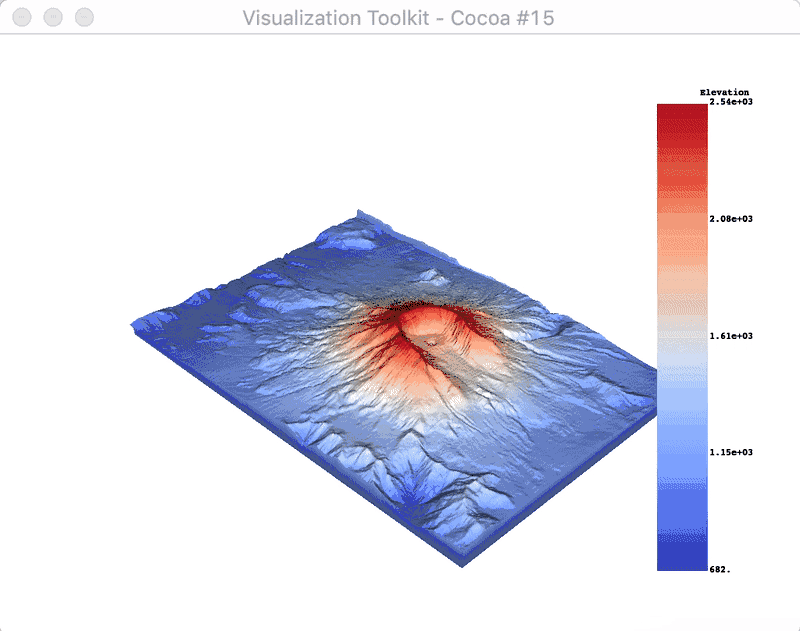# Customize Scalar Bars¶

Walk through of all the different capabilities of scalar bars and how a user can customize scalar bars.

```# sphinx_gallery_thumbnail_number = 2
import pyvista as pv
from pyvista import examples
```

By default, when plotting a dataset with a scalar array, a scalar bar for that array is added. To turn off this behavior, a user could specify `show_scalar_bar=False` when calling `.add_mesh()`. Let’s start with a sample dataset provide via PyVista to demonstrate the default behavior of scalar bar plotting:

```# Load St Helens DEM and warp the topography

# First a default plot with jet colormap
p = pv.Plotter()
# Add the data, use active scalar for coloring, and show the scalar bar
# Display the scene
p.show()
```We could also plot the scene with an interactive scalar bar to move around and place where we like by specifying passing keyword arguments to control the scalar bar via the `scalar_bar_args` parameter in `pyvista.BasePlotter.add_mesh()`. The keyword arguments to control the scalar bar are defined in `pyvista.BasePlotter.add_scalar_bar()`.

```# create dictionary of parameters to control scalar bar
sargs = dict(interactive=True)  # Simply make the bar interactive

p = pv.Plotter(notebook=False)  # If in IPython, be sure to show the scene
p.show()
# Remove from plotters so output is not produced in docs
pv.plotting._ALL_PLOTTERS.clear()
```Or manually define the scalar bar’s location:

```# Set a custom position and size
sargs = dict(height=0.25, vertical=True, position_x=0.05, position_y=0.05)

p = pv.Plotter()
p.show()
```The text properties of the scalar bar can also be controlled:

```# Controlling the text properties
sargs = dict(
title_font_size=20,
label_font_size=16,
n_labels=3,
italic=True,
fmt="%.1f",
font_family="arial",
)

p = pv.Plotter()
p.show()
```Labelling values outside of the scalar range

```p = pv.Plotter()
below_color='blue', above_color='red',
scalar_bar_args=sargs)
p.show()
```Annotate values of interest using a dictionary. The key of the dictionary must be the value to annotate, and the value must be the string label.

```# Make a dictionary for the annotations
annotations = {
2300: "High",
805.3: "Cutoff value",
}

p = pv.Plotter()